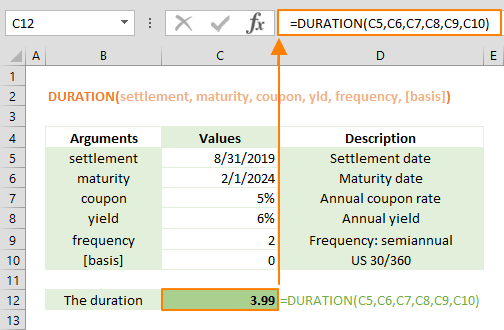The DURATION Excel function is a Financial formula that calculates and returns the annual duration of a security based on the Macauley duration formula. In this guide, we’re going to show you how to use the DURATION function and also go over some tips and error handling methods.

## Supported versions

• All Excel versions

## DURATION Excel Function Syntax

DURATION(settlement, maturity, coupon, pr, redemption, frequency, [basis])

## Arguments

 settlement The security's settlement date. The security settlement date is the date after the issue date when the security is traded to the buyer. maturity The security's maturity date. The maturity date is the date when the security expires. coupon The security's annual coupon rate. yld The security's annual yield. frequency The number of coupon payments per year. For annual payments, frequency = 1; for semiannual, frequency = 2; for quarterly, frequency = 4. [basis] Optional. The type of day count basis to use. 0 or omitted: US (NASD) 30/360 1: Actual/actual 2: Actual/360 3: Actual/365 4: European 30/360

## DURATION Excel Function Examples

The following example shows how to calculate the annual duration of a coupon purchased on August 8, 2019, with maturity date of February 2nd, 2024. The annual coupon rate is 5.0%  and the yield is 6.0%.  The payments will be made semiannually based on the US (NASD) 30/360 day count.## Tips

• Microsoft recommends using the DATE or other similar functions that can return a date serial number.
• Excel keeps date and time values as numbers. Excel assumes that Jan 1st, 1900 is 1, and every subsequent date value is based on this. While whole numbers represent days, decimals represent time values. For example; 1/1/2018 is equal to 43101, and 12:00 is equal to 5.
• Settlement, maturity, and basis are truncated to integers.
• Other price-related functions:
• PRICE returns the price per \$100 face value of a security that pays periodic interest.
• PRICEDISC returns the price per \$100 face value of a discounted security.
• PRICEMAT returns the returns the price per \$100 face value of a security that pays interest at maturity.
• YIELD returns yield on a security that pays periodic interest.

## Common Issues

• If settlement or maturity are not valid dates, the DURATION Excel function returns the #VALUE! error.
• The DURATION returns the #NUM! error value if coupon < 0 or if yld < 0.
• If [basis] < 0 or if [basis] > 4, DURATION returns the #NUM! error value.
• If settlementmaturity, DURATION returns the #NUM! error value.
• Settlement, maturity, frequency, and [basis] are truncated to integers.